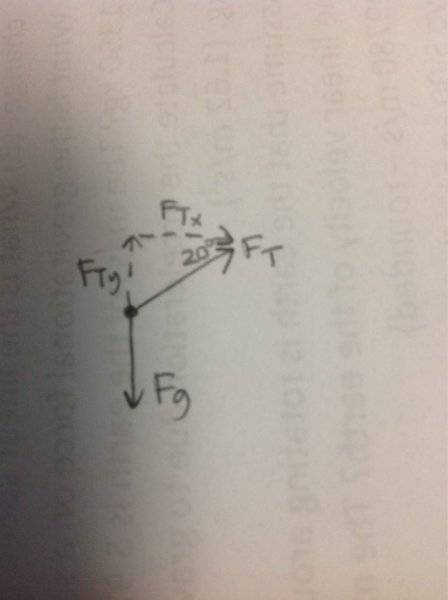# Circular Motion and Gravitation of tether ball

## Homework Statement

There is a tether ball swinging around in a uniform circle on a rope that is 1.90m long. Marcus hit the ball so hard that the string holding the ball made a 20.0 degree angle to the horizontal. What is the tension in the rope if the ball has a mass of 1.40 kg? Assume that the rope is not wrapping around pole so that the length of the rope doesn't change. What is the linear speed of the ball?
Show all Work

F=ma=Ft-Fg
Ftx=fgcos
Fty=fgsin

## The Attempt at a Solution

F=ma=Ft-Fg
Ftx=Fgcos?
Fty=Fgsin20=1.40*9.8(sin20)=4.69

I tried Ft=fg/sin20 and got 40.1, searched it on google, but I don't understand why i did Ft=fg/sin20, nor how i get it.

Chestermiller
Mentor
Have you drawn a free body diagram? What are the forces acting on the ball? If T is the tension in the rope, what is the component of the tension force in the vertical direction. What is the component of the tension force in the horizontal direction?

Chet

haruspex
Homework Helper
Gold Member
F=ma=Ft-Fg
As a vector equation, yes. But if you mean vectors it's better to indicate that, e.g. underlined, or in bold, or with arrows above.
Ftx=Fgcos?
Ftx is, I presume, the horizontal component of the tension. What is the horizontal component of the g force. Is there a horizontal acceleration?
Fty=Fgsin20
Same questions for vertical.

Thank you guys! I finally understand how to get the force of tension, but for velocity I am not sure which equation to use.

haruspex
Homework Helper
Gold Member
Thank you guys! I finally understand how to get the force of tension, but for velocity I am not sure which equation to use.
So what do you get for the radial acceleration?

A=f/m, so 40.1/1.4? Then that would be 28.6m/s^2
Would I use the equation a=mv^2/r? If yes then it would be 28.6=1.4v^2/1.9, v=6.23, but I was told the answer should be 6.93

haruspex
Homework Helper
Gold Member
A=f/m, so 40.1/1.4? Then that would be 28.6m/s^2
Would I use the equation a=mv^2/r? If yes then it would be 28.6=1.4v^2/1.9, v=6.23, but I was told the answer should be 6.93
Yes, 40.1 N is the tension. But the path is a horizontal circle, so what is the radial force?
This equation is wrong: a=mv^2/r. You have acceleration on the left and a force on the right.

Chestermiller
Mentor
The r in your equation should be 1.9 cos(20). Can you tell us why? What is the horizontal component of the tension force on the ball?

Would the equation be a=v^2/r?

I honestly don't understand how it works

haruspex
Homework Helper
Gold Member
Would the equation be a=v^2/r?
Yes.
I honestly don't understand how it works
The rope is inclined at an angle, but the circular path of the mass is in a horizontal plane. In order to analyse the motion as a circular orbit, we have to find the acceleration and radius in that plane. So the force leading to the acceleration is not the tension in the rope - it's only the horizontal component of that, and the radius is not the length of the rope - again, it's only the horizontal component of the length.
Did you draw a free body diagram? Can you post it or describe it?

Chestermiller
Mentor
I hate to say this, but this is what happens when the person trying to solve the problem does not make use of a free body diagram. SarahLee, Haruspex and I have both urged you to draw a free body diagram, and to use that diagram to help solve the problem. We are both very experienced at this, and yet we both always use free body diagrams. I can not emphasize how important it is to use free body diagrams. Has your professor not conveyed this to you?

Chet

I have drawn one, and I feel as though I understand how it works, but for me, I feel like the equations get me confused. I am only a sophomore in High School, and this is all very new to me.

haruspex
Homework Helper
Gold Member
I have drawn one, and I feel as though I understand how it works, but for me, I feel like the equations get me confused. I am only a sophomore in High School, and this is all very new to me.haruspex